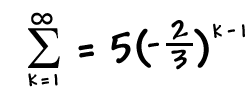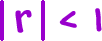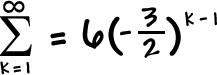Find the sumBut, we're not quite done yet.

 Our formula only works when.
 So, what if r is something like?  Then the series does not have a sum.  It shoots off to infinity.  When this happens, we call the series "divergent."

So, if someone asks you to find the sum of an INFINITE geometric sequence, CHECK THE RATIO!

 If, crunch the sum.
 If, say something like

"There's no finite sum.  This critter's divergent!"

TRY IT:

Find the sums (if possible):